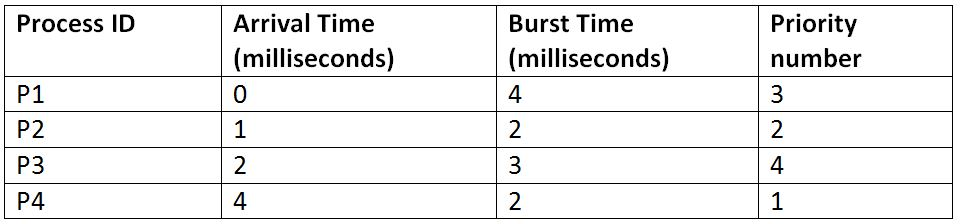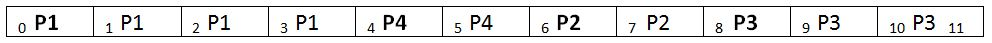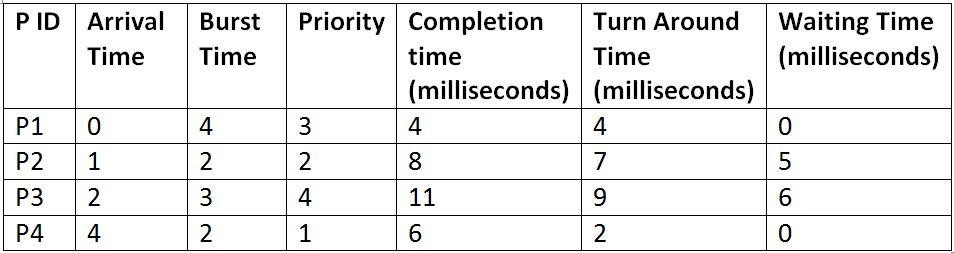# Priority Scheduling (Non preemptive) in Operating System

In this article, we are going to learn about priority scheduling, which is a type of process scheduling algorithms in the Operating systems. We will study only about its preemptive mode in this article. We will first describe how the processes are scheduled inside the system by using this algorithm and then will also look at an example for the further understanding of the entire working process of the operating system while following this scheduling algorithm.
Submitted by Monika Sharma, on June 24, 2019

Priority scheduling is a type of scheduling algorithm used by the operating system to schedule the processes for execution. The priority scheduling has both the preemptive mode of scheduling and the non-preemptive mode of scheduling. Here, we will discuss the non-preemptive priority scheduling algorithm.

As the name suggests, the scheduling depends upon the priority of the processes rather than its burst time. So, the processes, in this case, must also have the priority number in its details on the basis of which the OS will schedule it.

For example, suppose we have 4 processes: P1, P2, P3 and P4 and they enter the CPU as follows:

Note: Here, lower the priority number, higher is the priority.As per the non-preemptive priority scheduling, the processes will be executed as follows:

Gant Chart:Explanation:

• There is only P1 available at time 0, so it will be executed first irrespective of the priority, and it cannot be preempted in between before its completion.
• When it is completed at 4th-time unit, we have all P2, P3, and P4 available. So, they are executed according to their priorities.```    Total Turn Around Time = 4 + 7 + 9 + 2
= 22 milliseconds
Average Turn Around Time= Total Turn Around Time / Total No. of Processes
= 22 / 4
= 5.5 milliseconds

Total Waiting Time = 0 + 5 + 6 + 0
= 11 milliseconds
Average Waiting Time = Total Waiting Time / Total No. of Processes
= 11 / 4
= 2.75 milliseconds
```